Скачать презентацию Figure 7 1 The Nature of Waves 1

bca7bd6242c59fbd7edd205950f1f500.ppt

• Количество слайдов: 59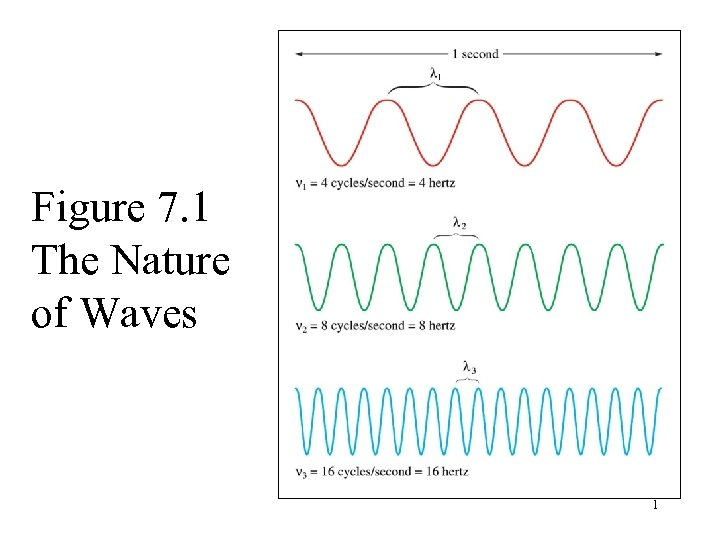Figure 7. 1 The Nature of Waves 1A Beautiful Rainbow 2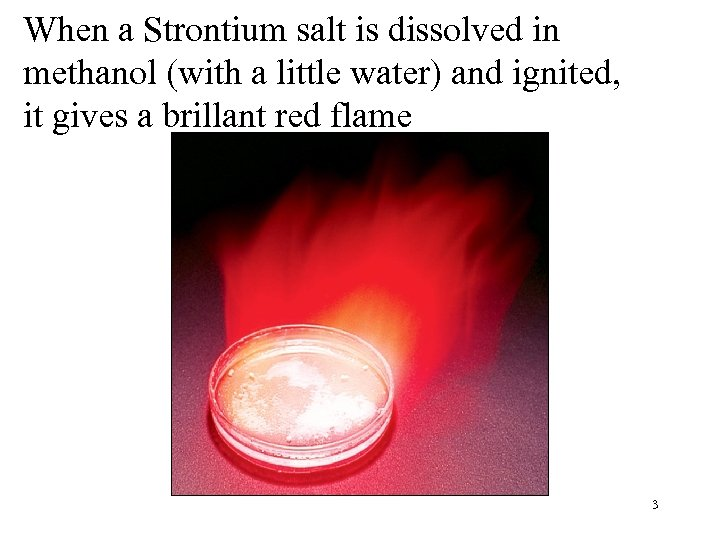When a Strontium salt is dissolved in methanol (with a little water) and ignited, it gives a brillant red flame 3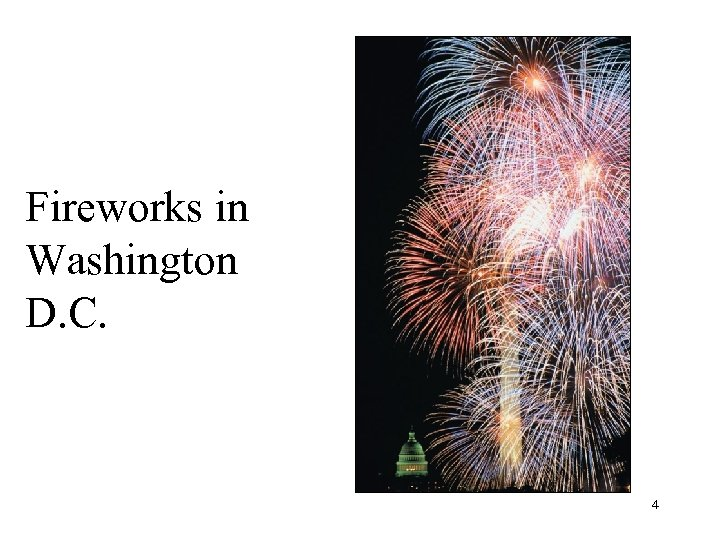Fireworks in Washington D. C. 4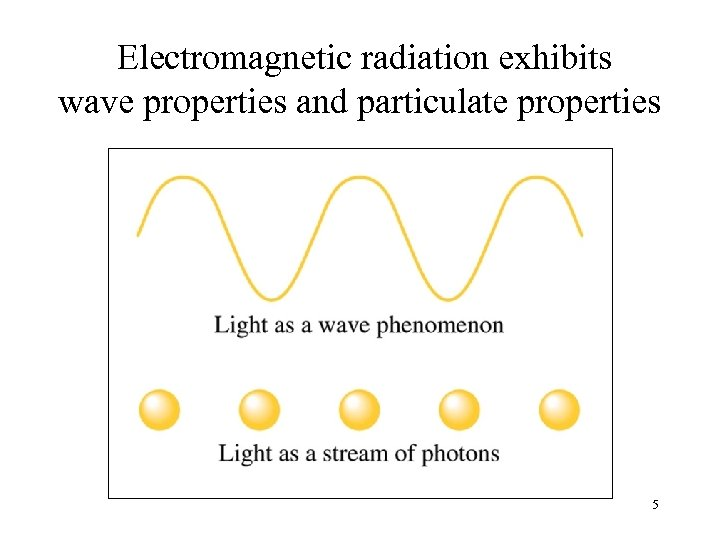Electromagnetic radiation exhibits wave properties and particulate properties 5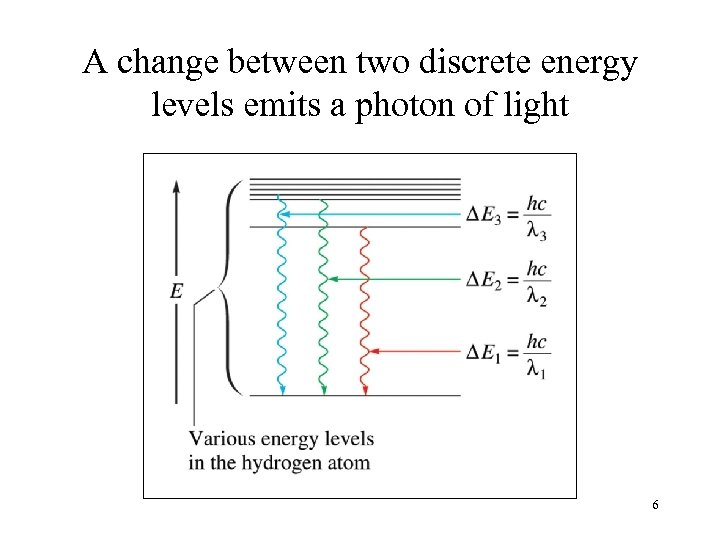A change between two discrete energy levels emits a photon of light 6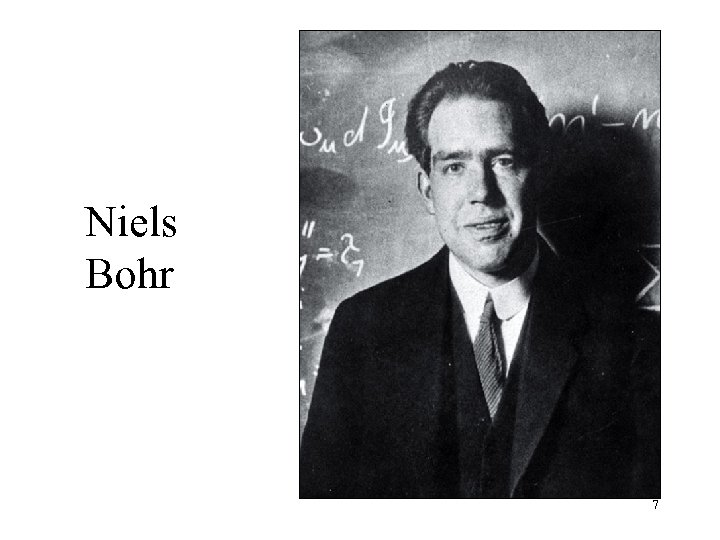Niels Bohr 7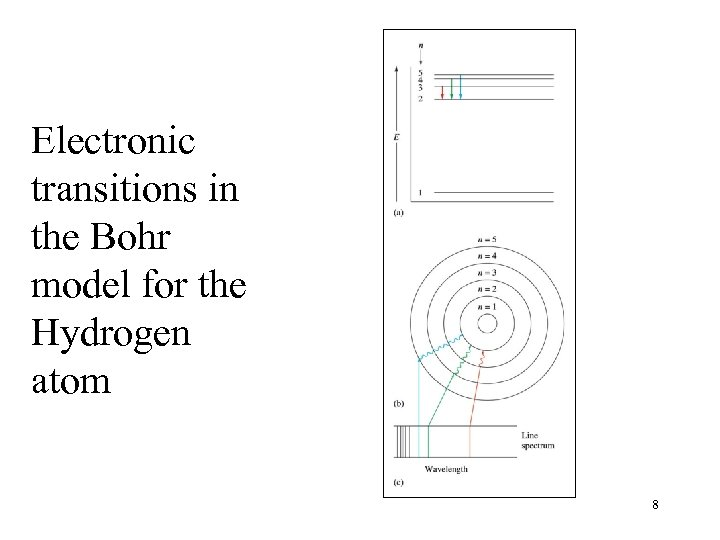Electronic transitions in the Bohr model for the Hydrogen atom 8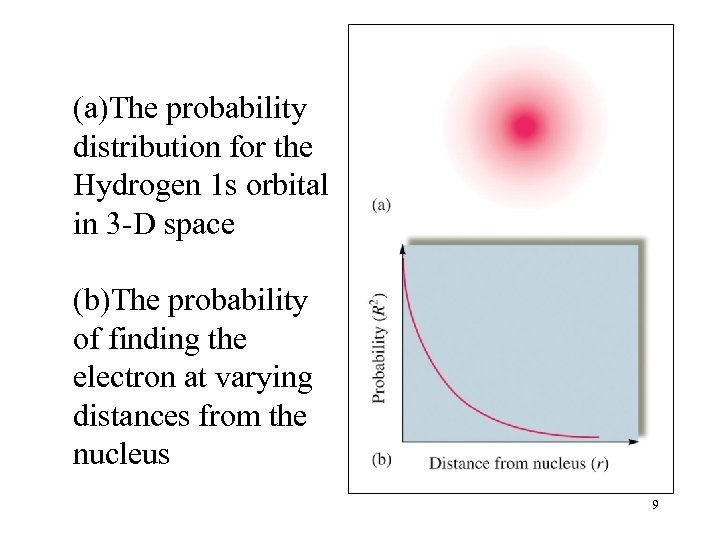(a)The probability distribution for the Hydrogen 1 s orbital in 3 -D space (b)The probability of finding the electron at varying distances from the nucleus 9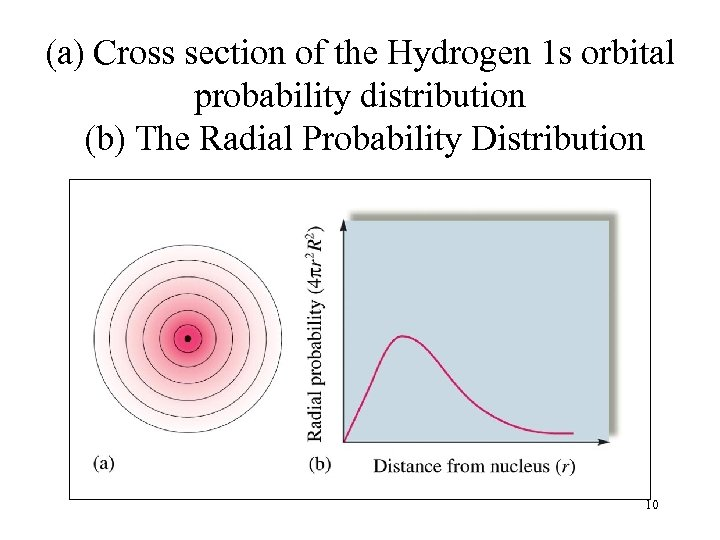(a) Cross section of the Hydrogen 1 s orbital probability distribution (b) The Radial Probability Distribution 10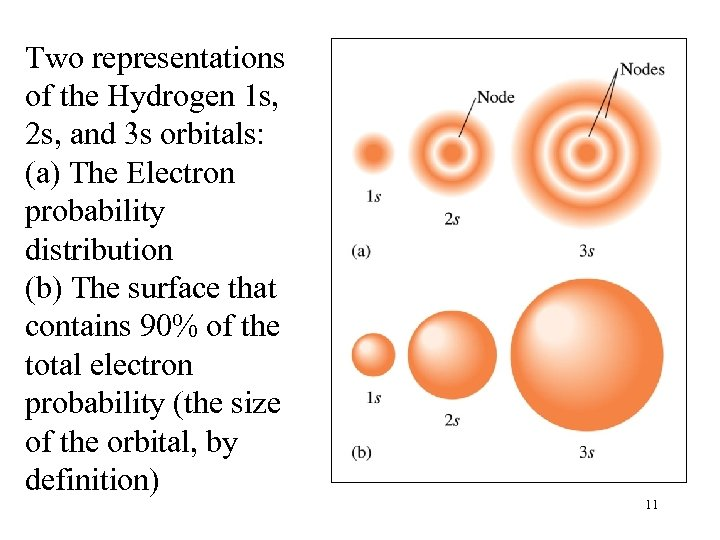Two representations of the Hydrogen 1 s, 2 s, and 3 s orbitals: (a) The Electron probability distribution (b) The surface that contains 90% of the total electron probability (the size of the orbital, by definition) 11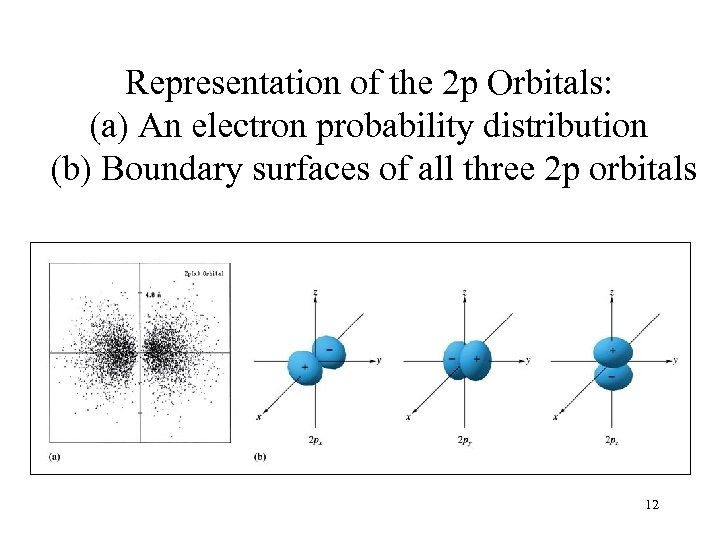Representation of the 2 p Orbitals: (a) An electron probability distribution (b) Boundary surfaces of all three 2 p orbitals 12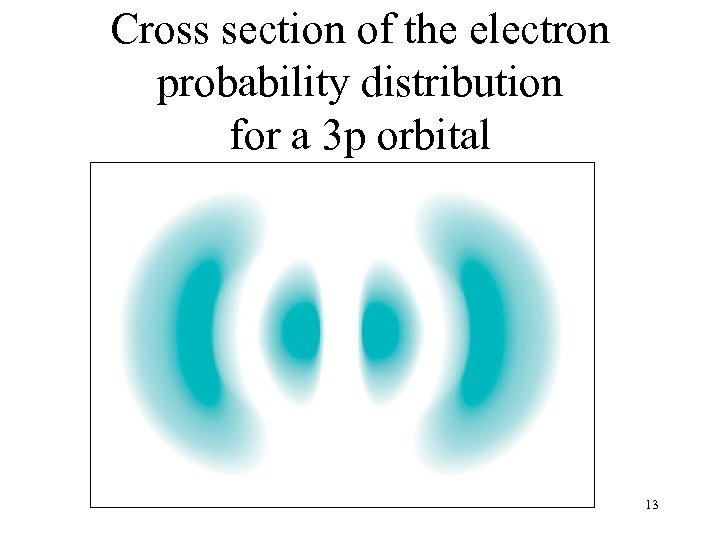Cross section of the electron probability distribution for a 3 p orbital 13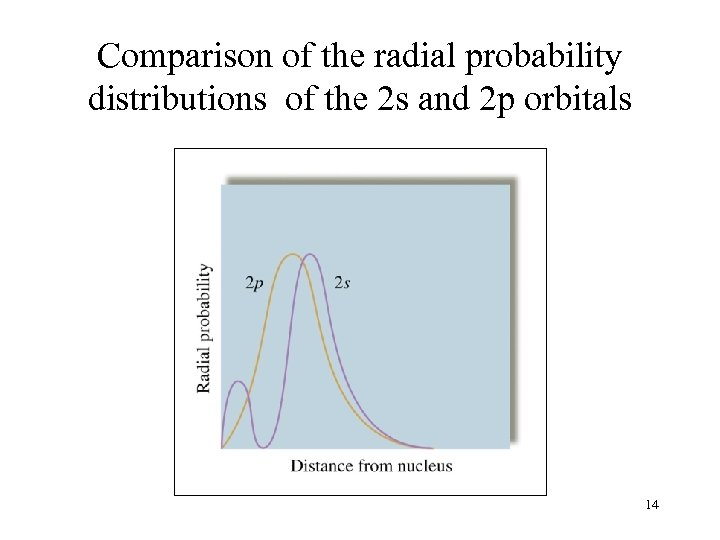Comparison of the radial probability distributions of the 2 s and 2 p orbitals 14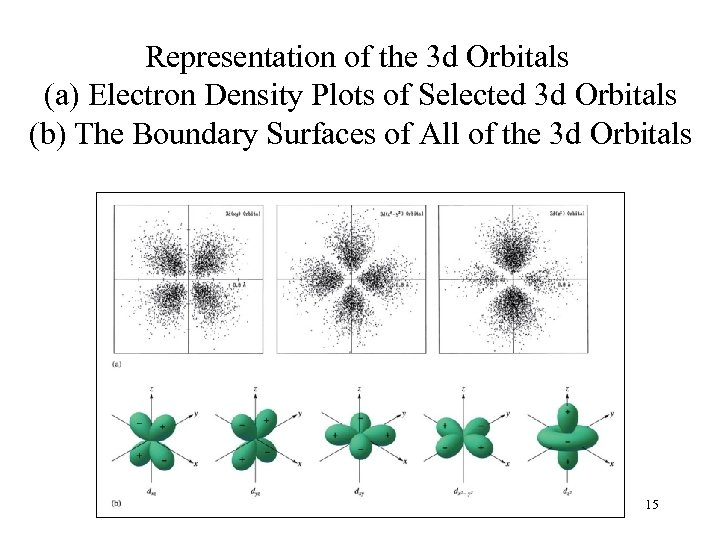Representation of the 3 d Orbitals (a) Electron Density Plots of Selected 3 d Orbitals (b) The Boundary Surfaces of All of the 3 d Orbitals 15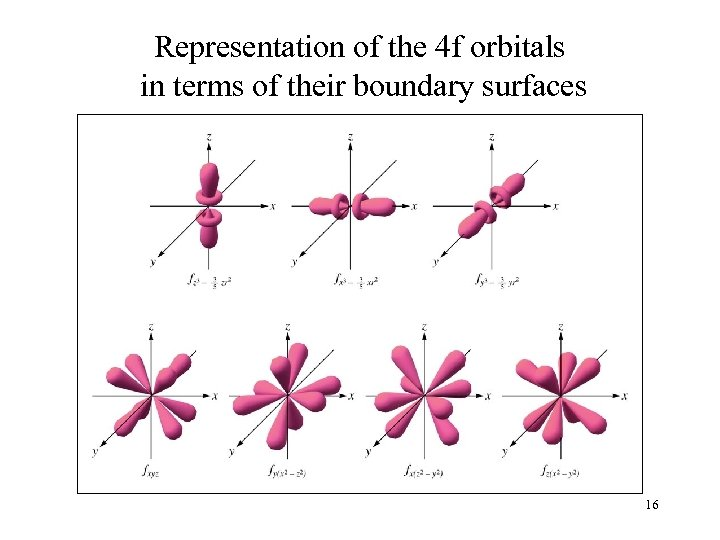Representation of the 4 f orbitals in terms of their boundary surfaces 16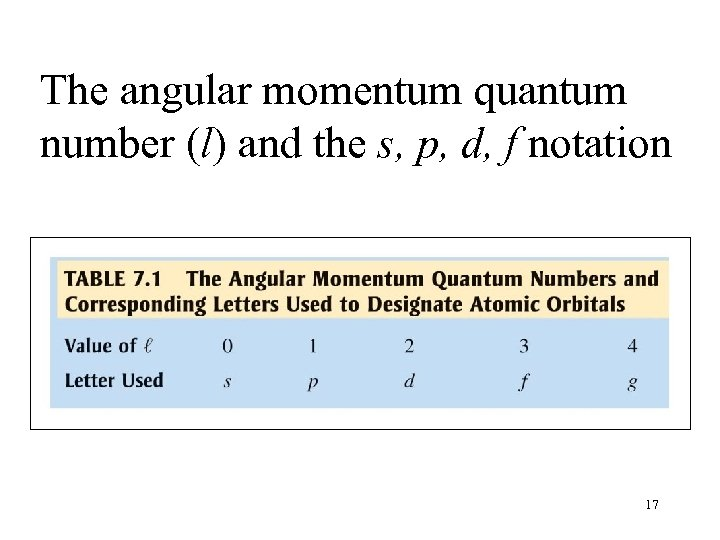The angular momentum quantum number (l) and the s, p, d, f notation 17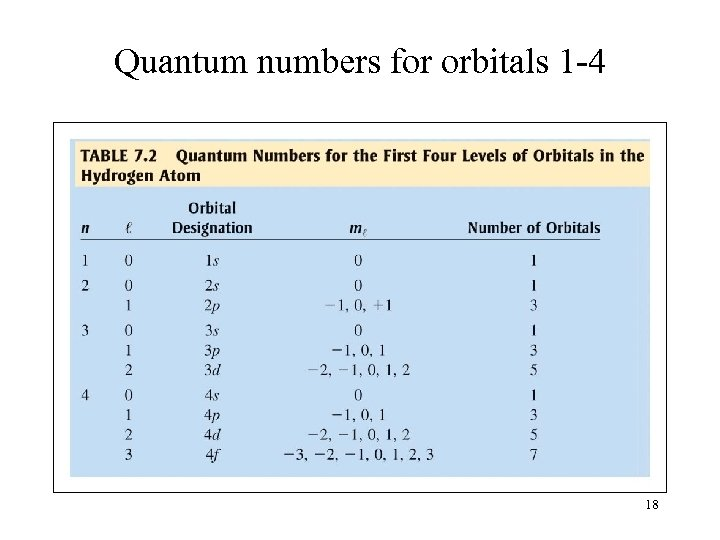Quantum numbers for orbitals 1 -4 18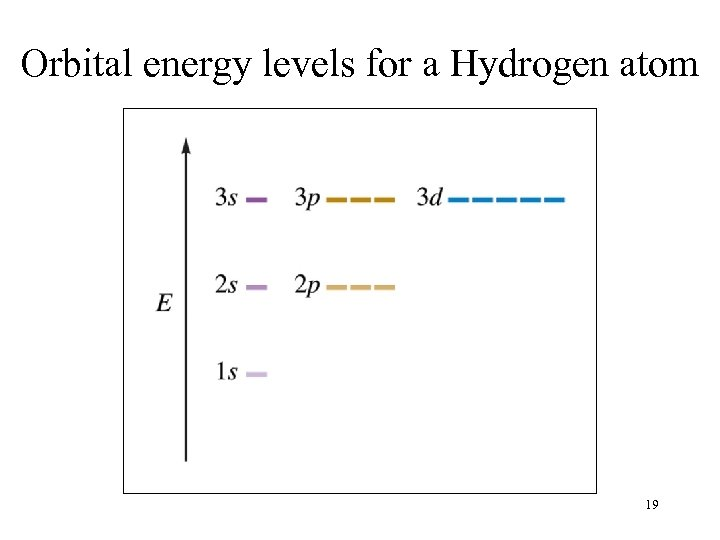Orbital energy levels for a Hydrogen atom 19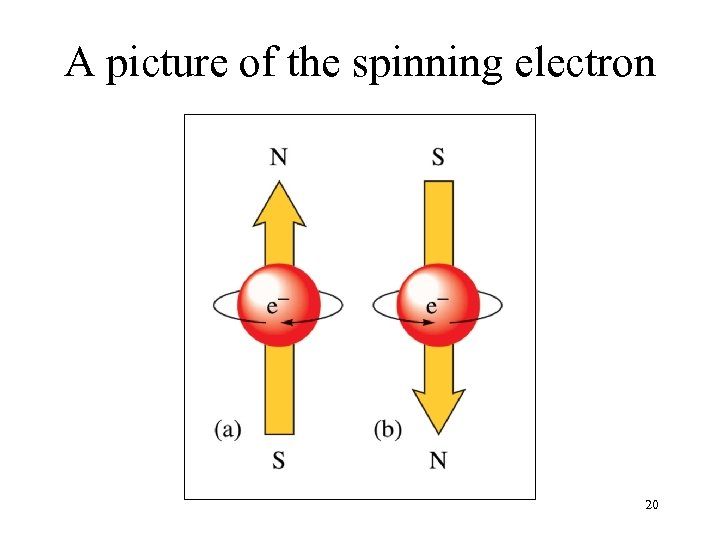A picture of the spinning electron 20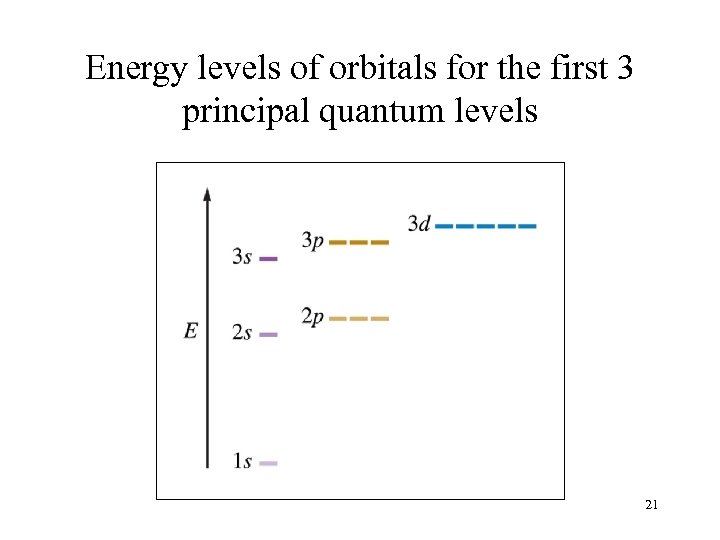Energy levels of orbitals for the first 3 principal quantum levels 21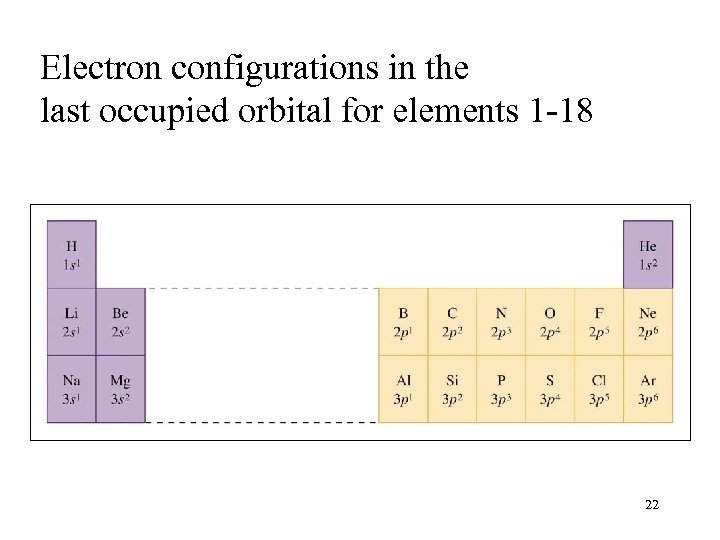Electron configurations in the last occupied orbital for elements 1 -18 22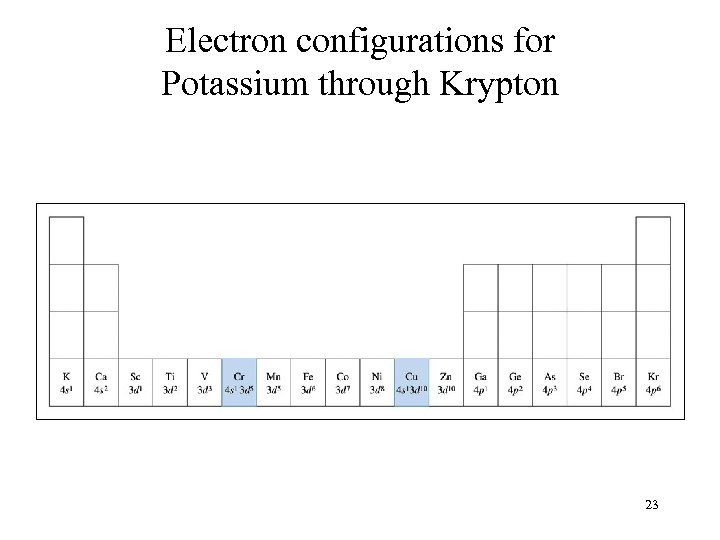Electron configurations for Potassium through Krypton 23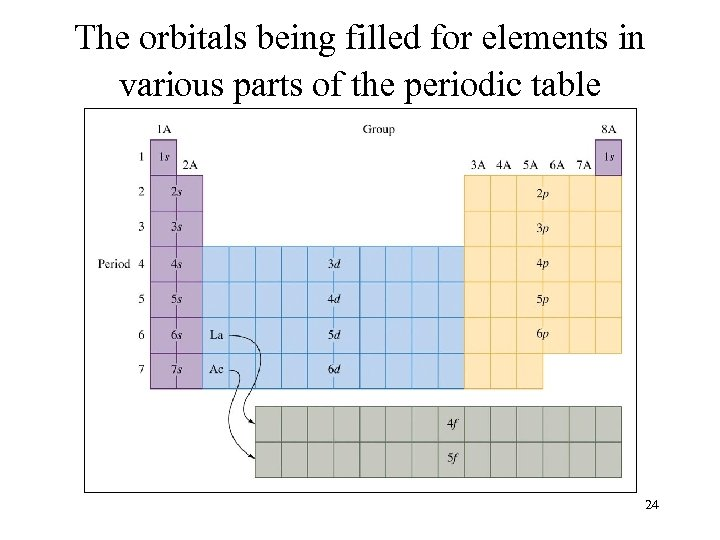The orbitals being filled for elements in various parts of the periodic table 24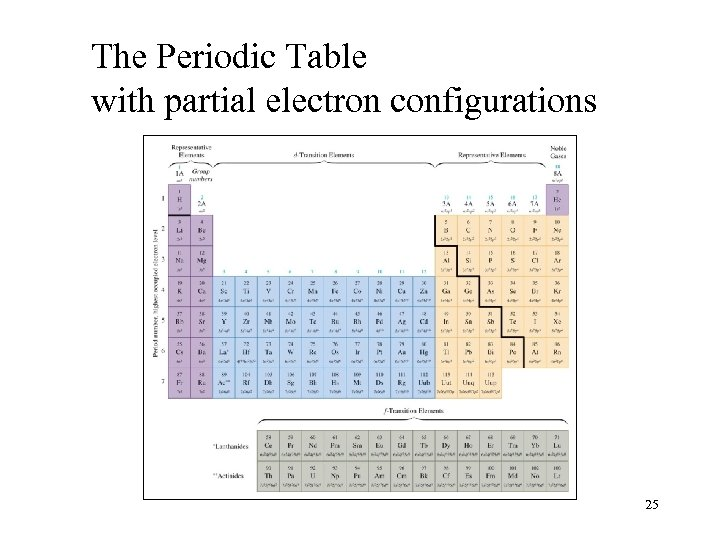The Periodic Table with partial electron configurations 25The End 2627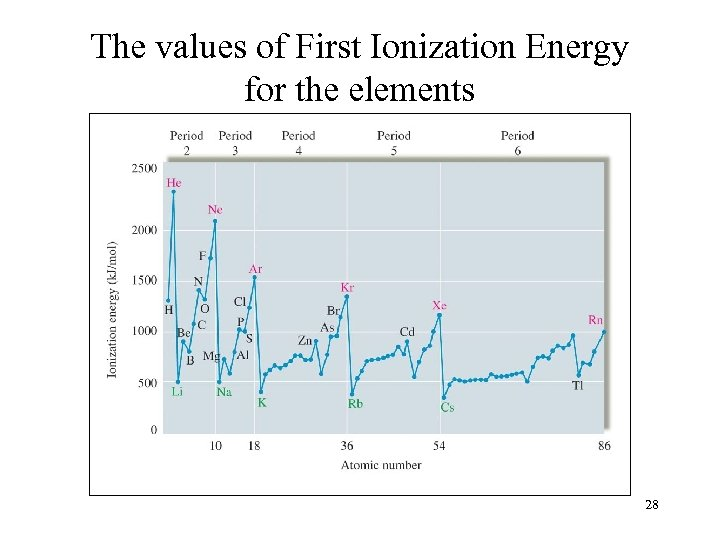The values of First Ionization Energy for the elements 28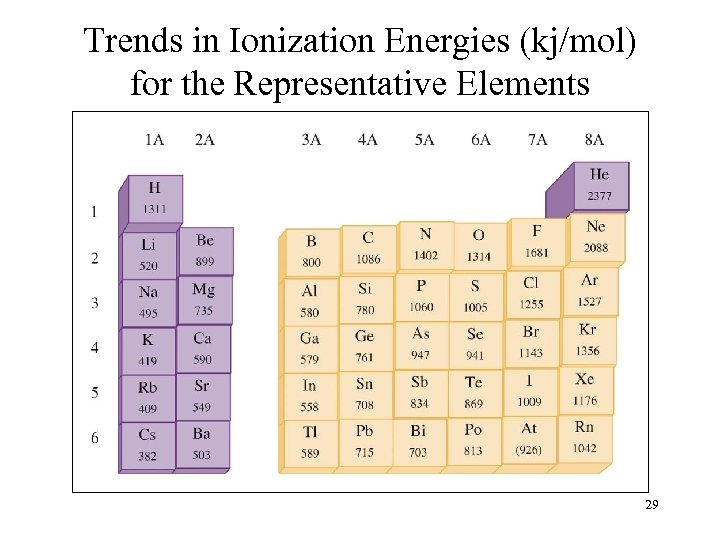Trends in Ionization Energies (kj/mol) for the Representative Elements 29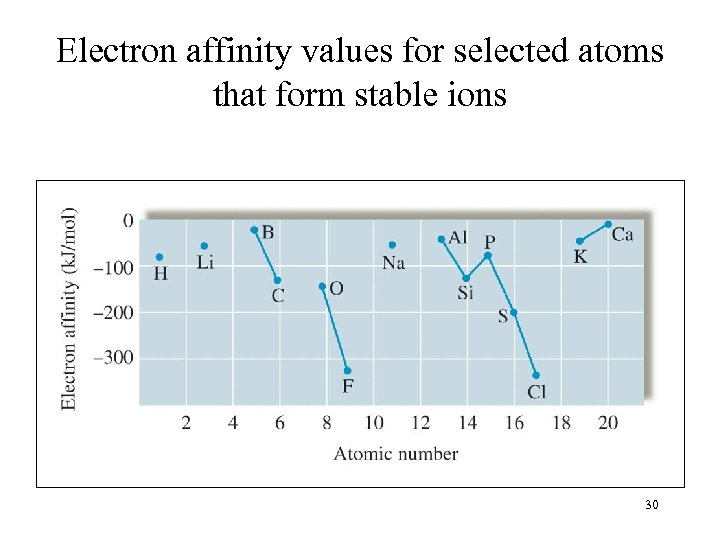Electron affinity values for selected atoms that form stable ions 30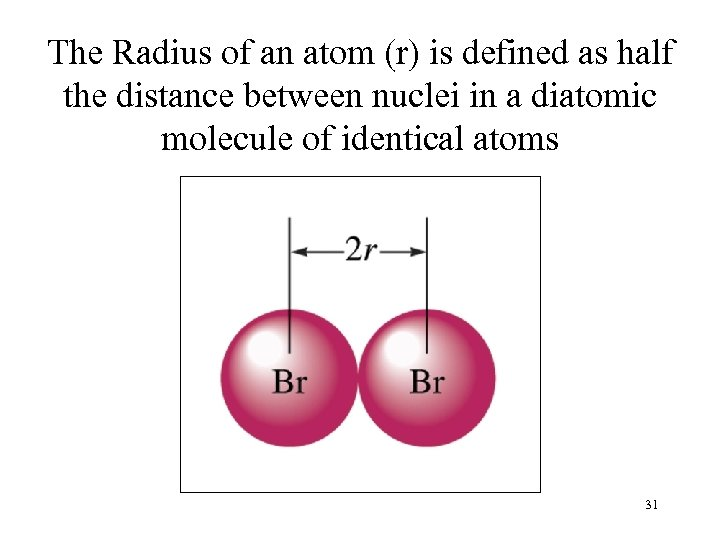The Radius of an atom (r) is defined as half the distance between nuclei in a diatomic molecule of identical atoms 31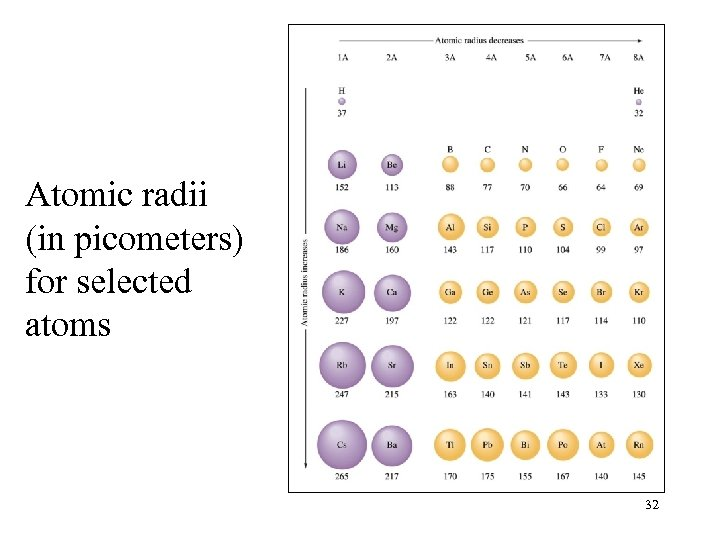Atomic radii (in picometers) for selected atoms 32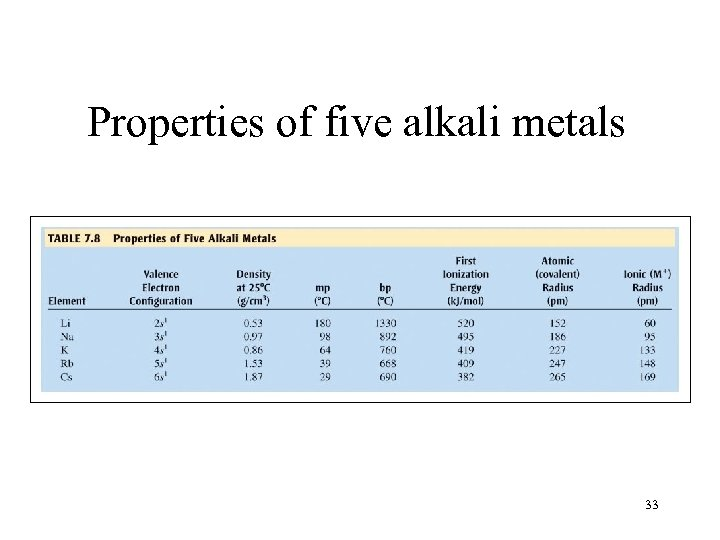Properties of five alkali metals 33First Ionization Energies for alkali metals and noble gases 34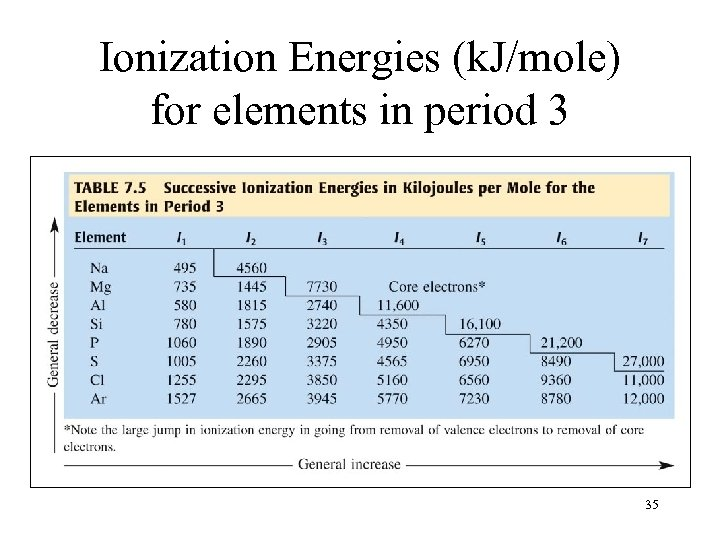Ionization Energies (k. J/mole) for elements in period 3 35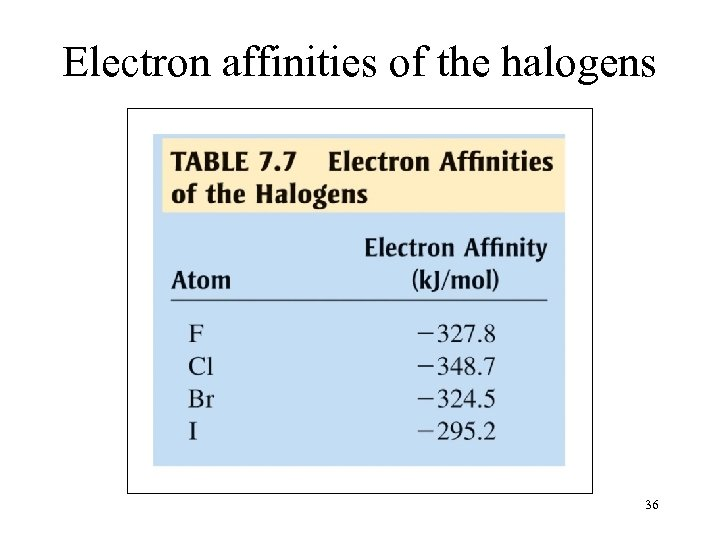Electron affinities of the halogens 36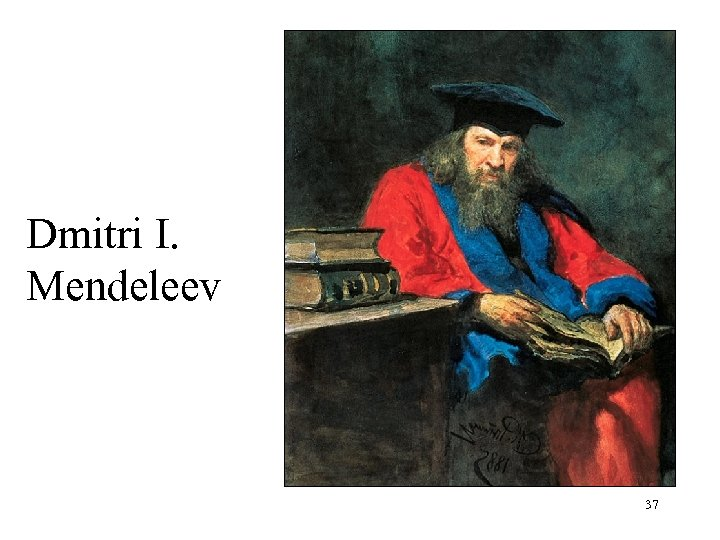Dmitri I. Mendeleev 37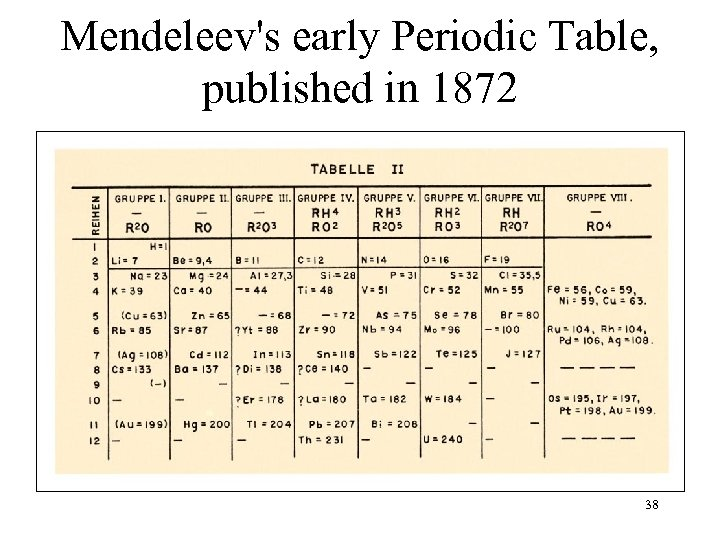Mendeleev's early Periodic Table, published in 1872 38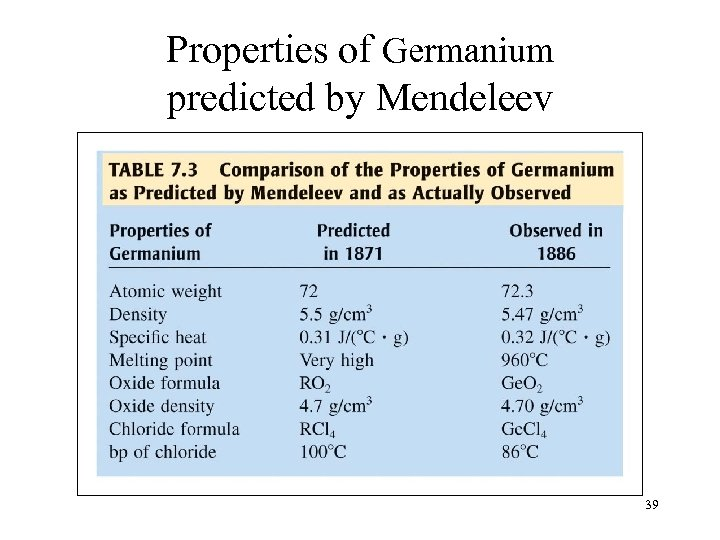Properties of Germanium predicted by Mendeleev 39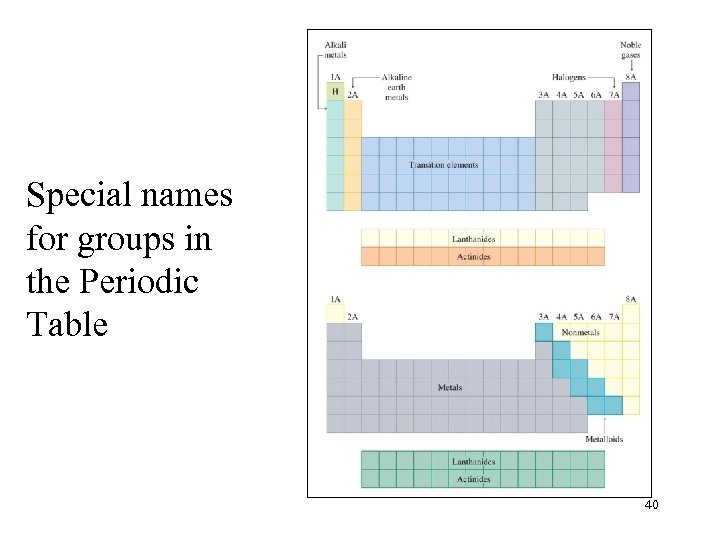Special names for groups in the Periodic Table 40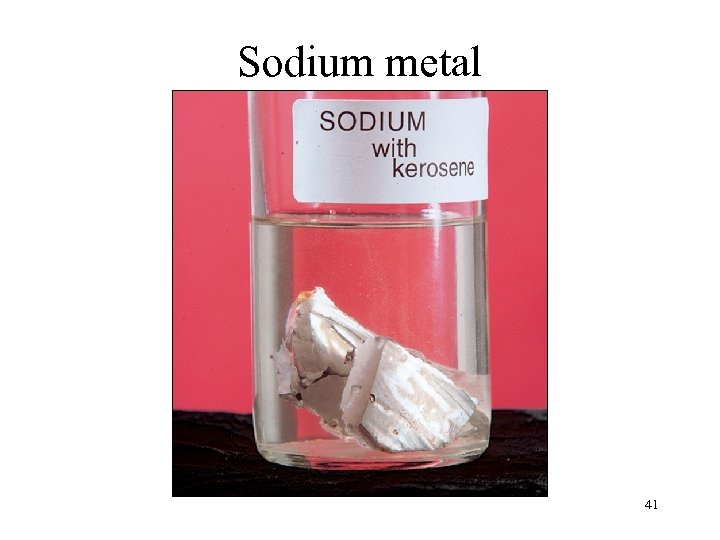Sodium metal 41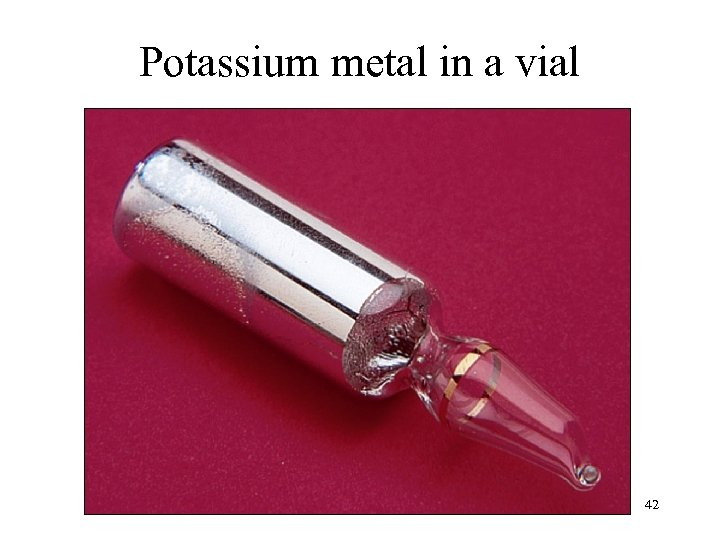Potassium metal in a vial 42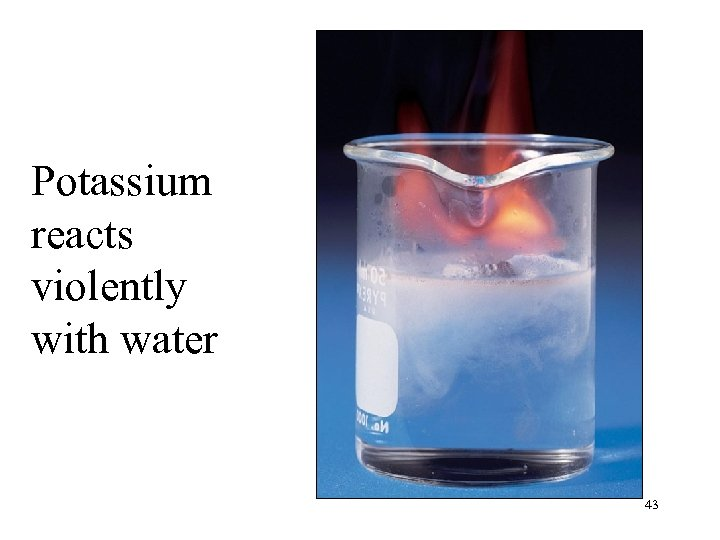Potassium reacts violently with water 43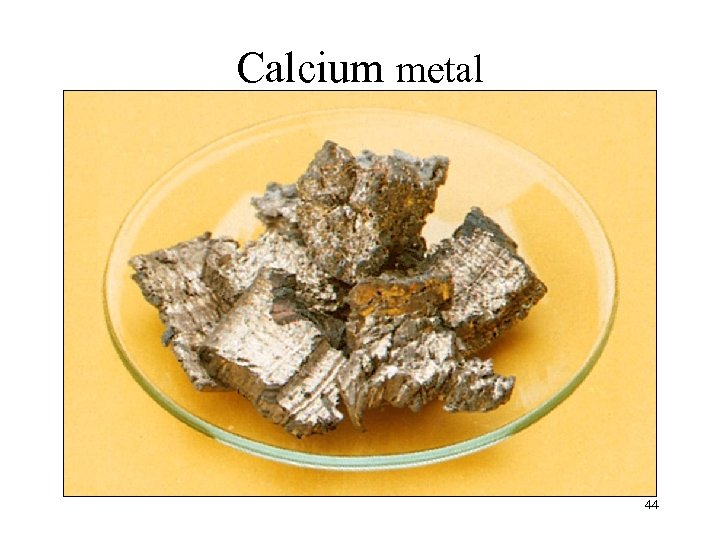Calcium metal 44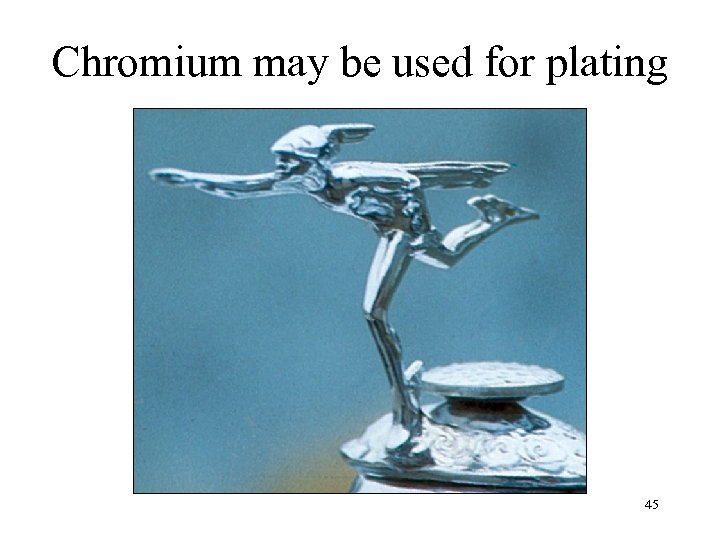Chromium may be used for plating 45Dr. Glenn Seaborg 46The End 4748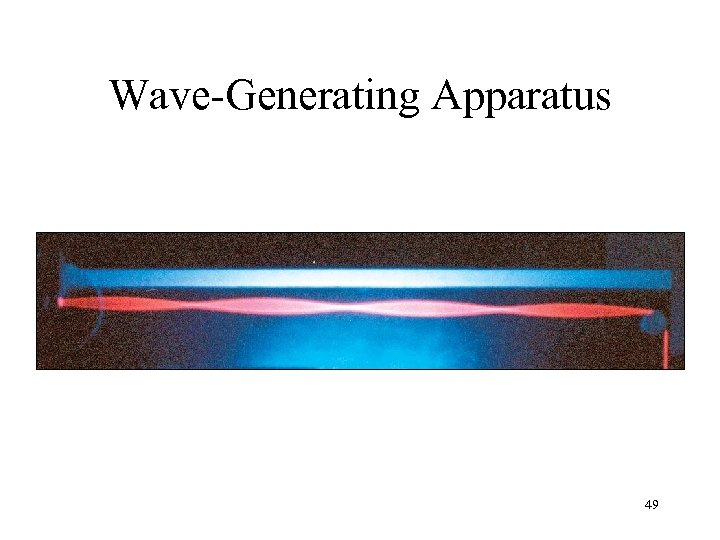Wave-Generating Apparatus 49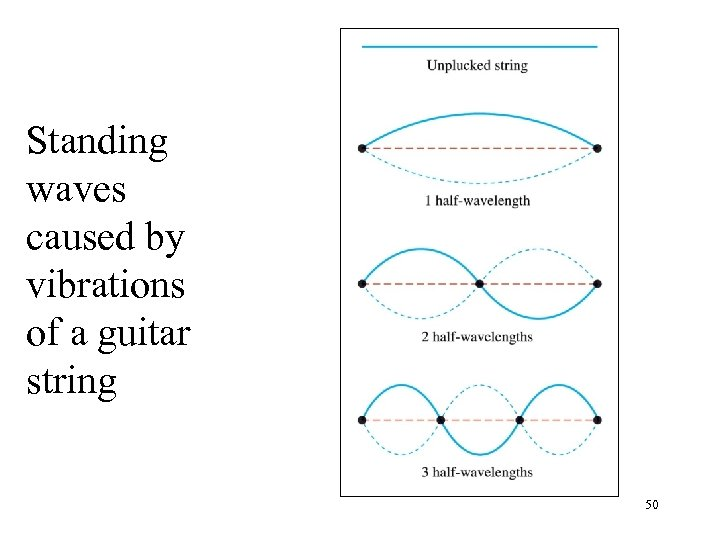Standing waves caused by vibrations of a guitar string 50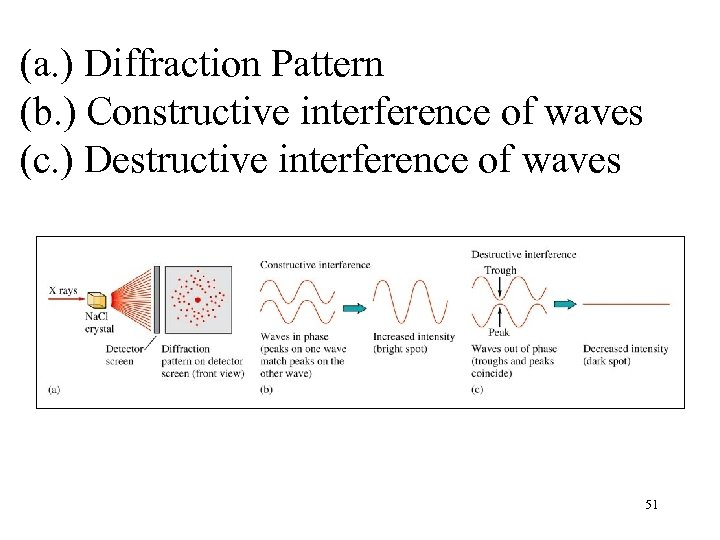(a. ) Diffraction Pattern (b. ) Constructive interference of waves (c. ) Destructive interference of waves 51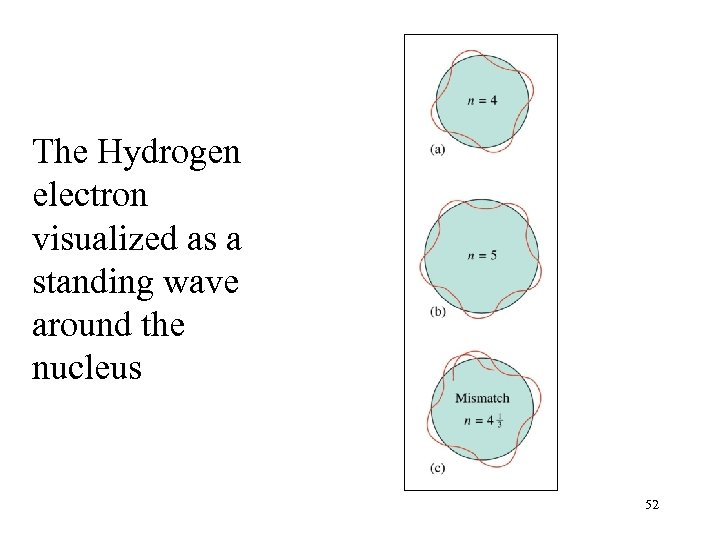The Hydrogen electron visualized as a standing wave around the nucleus 52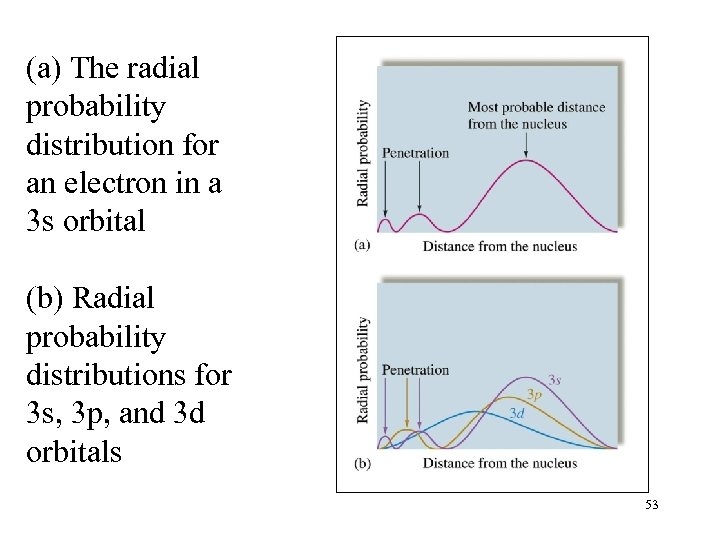(a) The radial probability distribution for an electron in a 3 s orbital (b) Radial probability distributions for 3 s, 3 p, and 3 d orbitals 53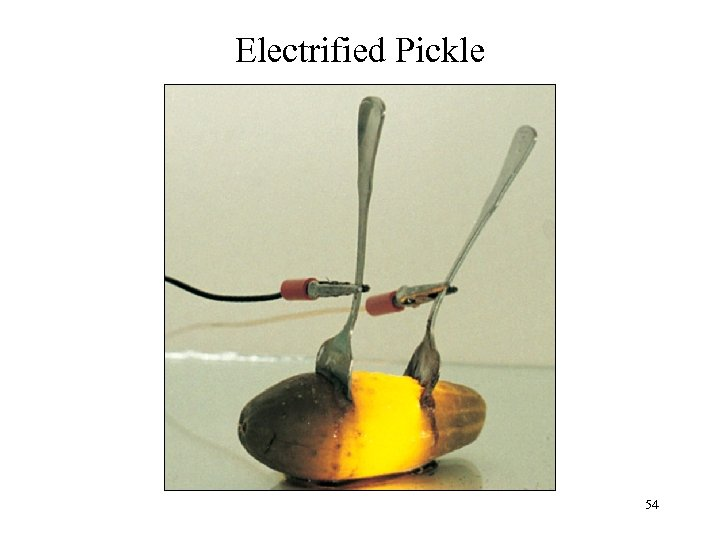Electrified Pickle 54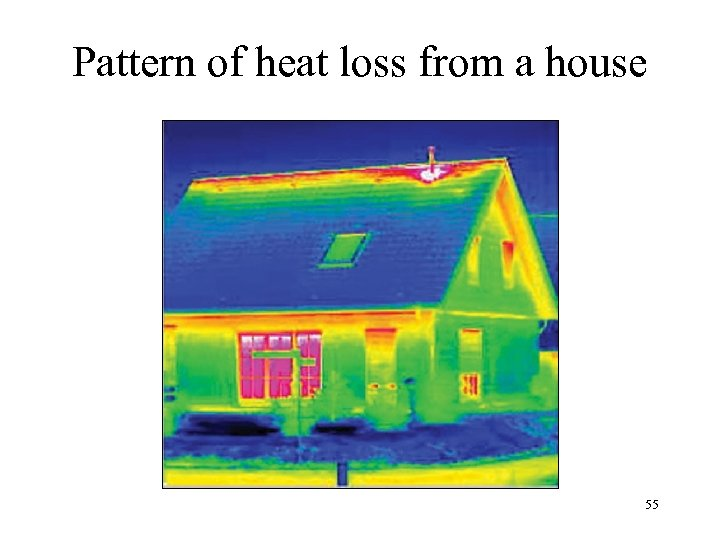Pattern of heat loss from a house 55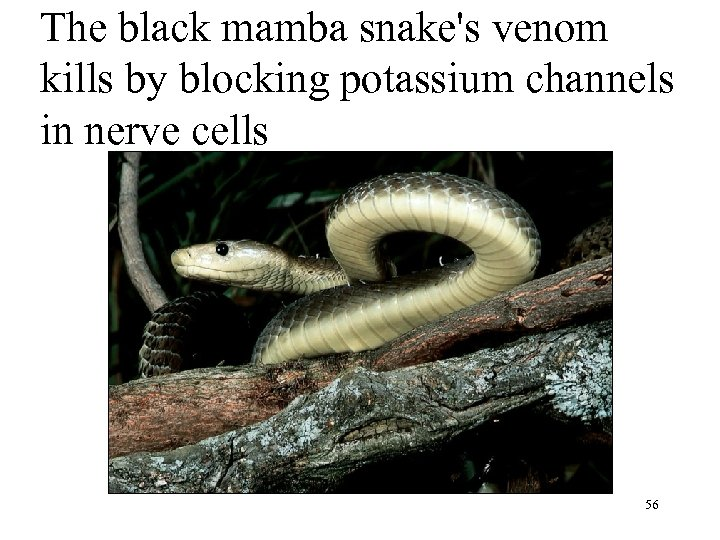The black mamba snake's venom kills by blocking potassium channels in nerve cells 56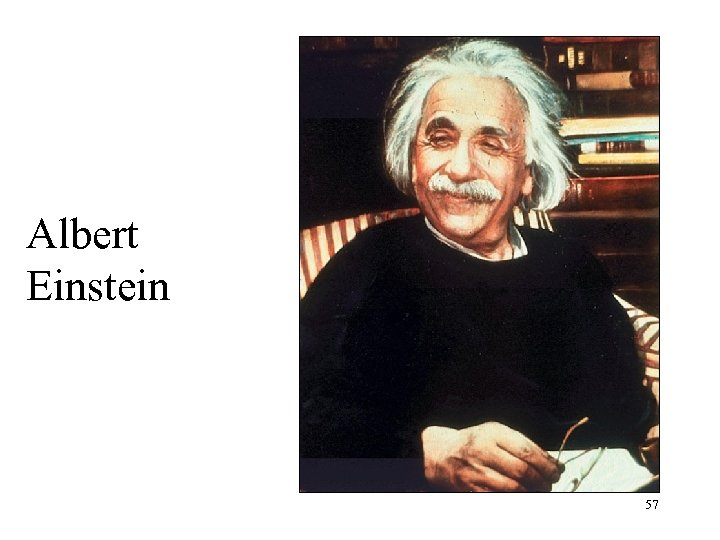Albert Einstein 57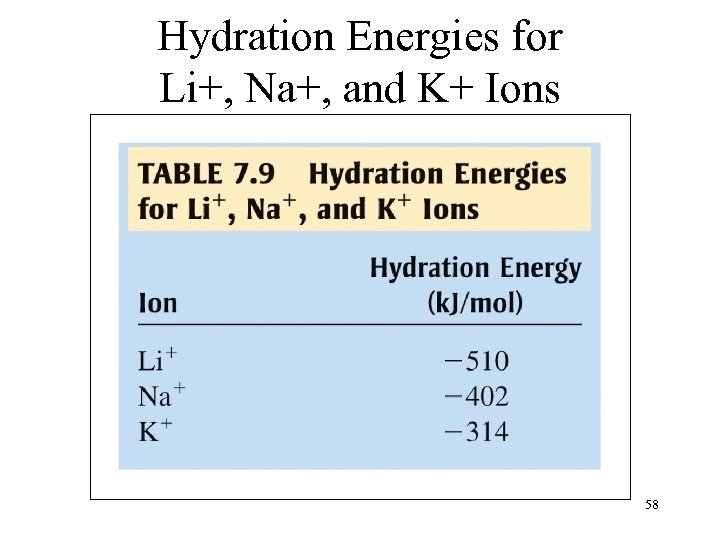Hydration Energies for Li+, Na+, and K+ Ions 58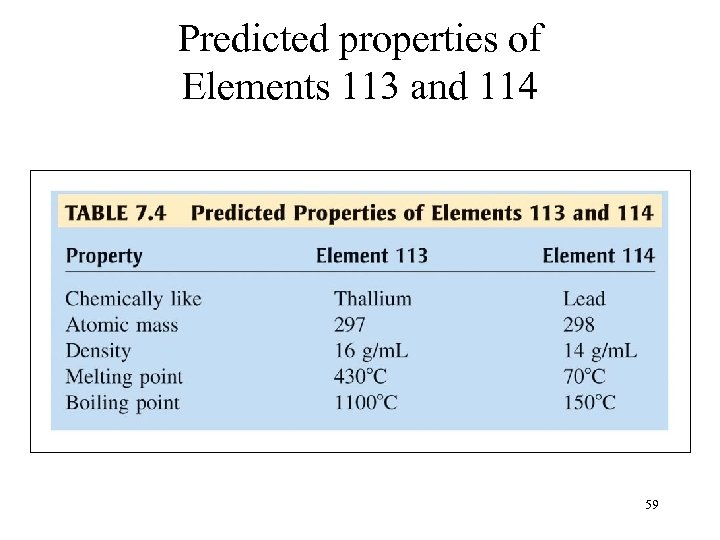Predicted properties of Elements 113 and 114 59